# Bamboo

Bamboo high 32 feet was at a certain height broken by the wind so the bamboo top reached the ground at a distance of 16 feet from the trunk. At what height from the ground was the bamboo broken?

x =  12

### Step-by-step explanation:

Try calculation via our triangle calculator.Did you find an error or inaccuracy? Feel free to write us. Thank you!Tips to related online calculators
Looking for help with calculating roots of a quadratic equation?
Do you have a linear equation or system of equations and looking for its solution? Or do you have a quadratic equation?
Pythagorean theorem is the base for the right triangle calculator.

#### You need to know the following knowledge to solve this word math problem:

We encourage you to watch this tutorial video on this math problem:

## Related math problems and questions:

• A mast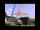A mast 32 meters high was broken by the wind so that its top touches the ground 16 meters from the pole. The still standing part of the mast, the broken part and the ground form a rectangular triangle. At what height was the mast broken?
• Broken treeThe tree is broken at 4 meters above the ground and the top of the tree touches the ground at a distance of 5 from the trunk. Calculate the original height of the tree.
• Broken treeThe tree was 35 meters high. The tree broke at the height of 10 m above the ground. Top but does not fall off it refuted on the ground. How far from the base of the tree lay its peak?
• Thunderstorm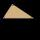The height of the pole before the storm is 10 m. After a storm when they come to check it they see that on the ground from the pole blows part of the column. Distance from the pole is 3 meters. At how high was the pole broken? (In fact, a rectangular tria
• The ropeA 68-centimeter long rope is used to make a rhombus on the ground. The distance between a pair of opposite side corners is 16 centimeters. What is the distance between the other two corners?
• Michael 2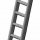Michael has a 35 foot ladder leaning against the side of his house. If the bottom of the ladder is 21 feet away from his house, how many feet above the ground does the ladder touch the house?
• A bridgeA bridge over a river has the shape of the arc with bases of the bridge at the river's edge. At the center of the river, the bridge is 10 feet above the water. At 27 feet from the edge of the river, the bridge is 9 feet above the water. How wide is the ri
• Pile of sandA large pile of sand has been dumped into a conical pile in a warehouse. The slant height of the pile is 20 feet. The diameter of the base of the sandpile is 31 feet. Find the volume of the pile of sand.
• Wind drift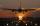The plane flies at 900 km/h, passing distance 1800 kilometers with the wind and once again against the wind for 4 h 1 min. What is the wind speed?
• Speed of Slovakian trainsRudolf decided to take the train from the station 'Ostratice' to 'Horné Ozorovce'. In the train timetables found train Os 5409 : km 0 Chynorany 15:17 5 Ostratice 15:23 15:23 8 Rybany 15:27 15:27 10 Dolné Naštice 15:31 15:31 14 Bánovce nad Bebravou 15:35 1
• Area of iso-trapFind the area of an isosceles trapezoid if the lengths of its bases are 16 cm and 30 cm, and the diagonals are perpendicular to each other.
• Isosceles triangleIn an isosceles triangle ABC with base AB; A [3,4]; B [1,6] and the vertex C lies on the line 5x - 6y - 16 = 0. Calculate the coordinates of vertex C.
• The Eiffel Tower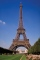The top of the Eiffel Tower is seen from a distance of 600 meters at an angle of 30 degrees. Find the tower height.
• Right triangleCalculate the missing side b and interior angles, perimeter, and area of ​​a right triangle if a=10 cm and hypotenuse c = 16 cm.
• TangentsTo circle with a radius of 41 cm from the point R guided two tangents. The distance of both points of contact is 16 cm. Calculate the distance from point R and circle centre.
• The base 2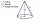The base diameter of a right cone is 16cm and it's slant height is 12cm. A. ) Find the perpendicular height of the cone to 1 decimal place. B. ) Find the volume of the cone, convert to 3 significant figure. Take pi =3.14
• TriangleCalculate the triangle sides if its area S = 630 and the second cathetus is shorter by 17.